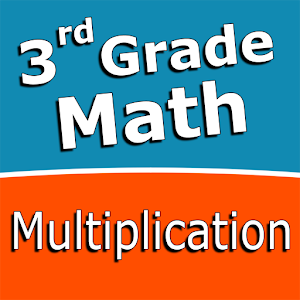Türkçe

Her gün ücretsiz lisanslı Android uygulamaları ve oyunlar sunuyoruz.\$2.49
SÜRESI DOLMUŞ

# Android Giveaway of the Day - Third grade Math - Multiplication

Practice and improve the math skills!
\$2.49 SÜRESI DOLMUŞ
Kullanıcı puanı: 1 (100%) 0 (0%)

Bu programın süresi doldu. Third grade Math - Multiplication artık firmadan temin edilebilir.

Intuitive interface powered by handwriting input and a fun and engaging mini game in addition to a regular math trainer mode make our app stand out from the crowd of generic math learning apps.

With Third Grade Math - Multiplication you can practice and improve the following math skills:
- Multiplication tables for 2, 3, 4, 5, 10
- Multiplication tables for 6, 7, 8, 9
- Multiplication tables up to 10×10
- Multiplication tables up to 12×12
- Multiply by a multiple of ten
- Multiply one-digit numbers by two-digit numbers
- Multiply one-digit numbers by three-digit numbers
- Multiply three 1-digit numbers
- Multiply numbers ending in zeroes

Choose between two different modes:
- Math Trainer
- Math Shot mini-game

Math Trainer mode helps you to master the math facts, you can solve math problems at you own pace without any time constrain.

Math Shot is a math mini-game with fun and engaging game-play and as we all know learning through play and fun is more effective. The game difficulty adapts to player's skills and makes the game suitable for all ages. Math Shot helps students to develop math fact fluency - the result of consistent learning, repetition, and practice.

Sergey Malugin

Education

5.0.0

15M

Everyone

4.0 and up

## Third grade Math - Multiplication üzerine yorumlar

Thank you for voting!